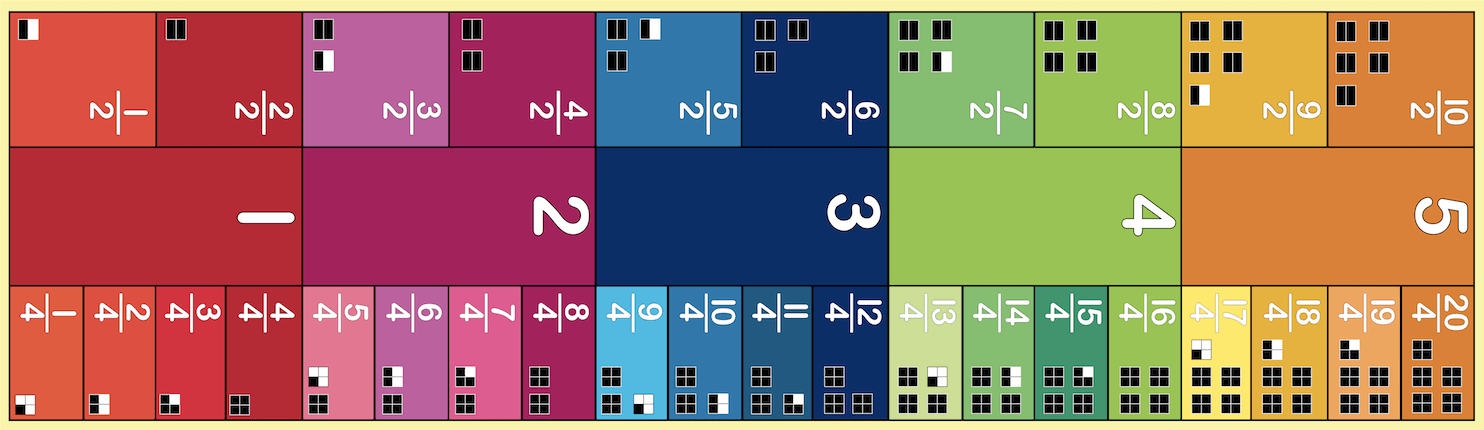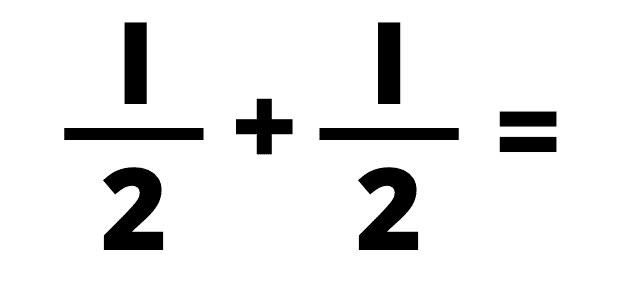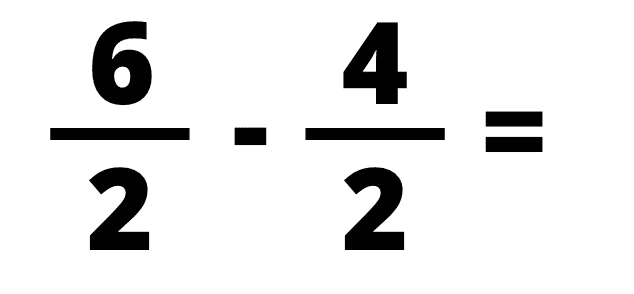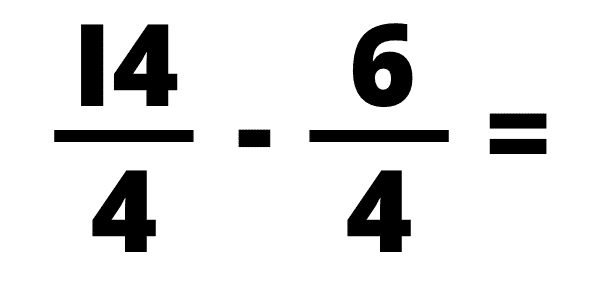## Fraction Walk Floor Mat (Halves & Quarters)The Fraction Walk (Halves & Quarters) Floor Mat offers a kinesthetic opportunity for students to learn fractions, equivalents fractions, fractions greater than one, and problems dealing with fractions.

Have your students start with the Pre-Test below. Then, spend some time jumping on the mat, using the activities below to guide you!

## Before hopping on the mat:

Ask your child how many of these by problems they know. (Mark it off and try to remember for the final survey!)

How many halves are in 4?

How many quarters are in 2?

How many halves are in 3?

How many quarters are in 5?## NOW, LET'S TRY SOME ACTIVITIES!

How many halves in five?

Have your student count how many halves are in five by stepping on each half while counting their steps from one to ten. If your student’s counting is out of sync with their steps, have them go back and start over. To help them emphasize each whole number, you can have them hop to the middle box after reaching the fraction that equals a whole. The student hops on 1⁄2 and says, “one half.” The student hops on 2/2 and says, “two halves equal one whole” as they hop to the one. Continue with this pattern until they reach five.

Knowledge of skip counting helps students understand fractions. If your student knows how to skip count by twos, and they can see that there are two halves in each of the whole numbers, then they can skip count to figure out how many halves are in the other numbers on the mat. To figure out how many halves are in three, have your student count 2-4-6. To figure out how many halves are in five, count 2-4-6-8-10.To figure out how many quarters are in quarters, count 4-8-12-16-20. When students become solid with multiplication, they can multiply to find the number of halves in any whole number. For example, to figure out how many halves are in four, they multiply two times four. To figure out how many quarters are in five, multiply four times five.

Have them do the same activity with fourths once they are comfortable with halves.

Have your student start on START HERE. Have the student say “one half” while hopping with one foot in the 1/2 box. Then have the student jump (two feet) on the 2/2, clap and say, “two halves.” Continue alternating between one foot and two-footed movements to the end of the mat.

Repeat activity for quarters. Be sure that your student says the fractions correctly. Tell your student the fractions if your student doesn’t know them.

Have a student select a fraction and stand on it. Then, have the student hop on all of its equivalent fractions. The activity should go on until the student has reached all fractions equivalent to the one on which they started. Then, have them select another fraction or have the next student take a turn.

Students might not realize right away that the colors/shades correspond with equivalent fractions. If they do not pick up on it, ask them to look closer at the colors on the mat and see if they can find the pattern.

This floor mat offers a visual opportunity for students to understand the relationships between fractions greater than one and mixed numbers.

For example, to have your student figure out the mixed number equivalent to 7/2, ask your student, “How many whole numbers are in 7/2?” Have your student step on 7/2. Ask how many whole numbers that they can see on the mat before 7/2? Ask how many fraction pieces are left over. Have your student count the halves after the whole number three up to 7/2. Your student will be able to count “one half” Then your student will see that the improper fraction 7/2 is the same as three and one half (1⁄2).

On index cards, write +1/2, +3/2, etc. Have your student select a number on the mat to stand on. Have your student select a card from a large hat or box. Have the student walk on the mat as they add or subtract depending on the card they selected.

## After hopping on the mat:

Ask your child how many of these by problems they know now!